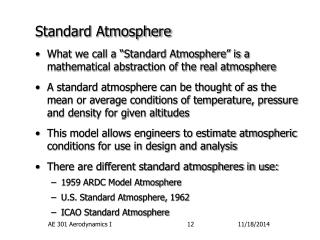DownloadDownload PresentationStandard Atmosphere

# Standard Atmosphere

Download Presentation## Standard Atmosphere

- - - - - - - - - - - - - - - - - - - - - - - - - - - E N D - - - - - - - - - - - - - - - - - - - - - - - - - - -
##### Presentation Transcript

1. Standard Atmosphere • What we call a “Standard Atmosphere” is a mathematical abstraction of the real atmosphere • A standard atmosphere can be thought of as the mean or average conditions of temperature, pressure and density for given altitudes • This model allows engineers to estimate atmospheric conditions for use in design and analysis • There are different standard atmospheres in use: • 1959 ARDC Model Atmosphere • U.S. Standard Atmosphere, 1962 • ICAO Standard Atmosphere AE 301 Aerodynamics I

2. Standard Atmosphere (continued) • Need to define what we mean by altitude first • Absolute Altitude, hA, is the height above the earth’s center • Geometric Altitude, hG, is the height above sea level. • Geopotential Altitude, h • A mathematical definition: the altitude for a given condition (T,p and r), if the gravitation acceleration was constant at the sea level value. • By hydrostatics: dp = -r g dhG or dp = -r go dh • Knowing the variation of g with hG yields the relation • Very little difference at normal airplane altitudes • We will use geopotential altitude almost exclusively!! AE 301 Aerodynamics I

3. 105 100 100 225.66 K Thermosphere 90 a4 = 4 X 10-3 K/m 80 80 79 ae = -4.5 X 10-3 K/m 165.66 K Mesosphere 60 60 53 Altitude, km Altitude, km Ozone 47 40 40 282.66 K Stratosphere a2 = 3 X 10-3 K/m 25 20 20 a1 = -6.5 X 10-3 K/m 11 Troposphere 288.16 K 216.66 K 0 0 160 160 200 200 240 240 280 280 320 320 Temperature, K Temperature, K Standard Atmosphere (continued) Physical Atmosphere Standard Atmosphere AE 301 Aerodynamics I

4. Standard Atmosphere (continued) • Mathematical Model • The standard atmosphere defines the temperature variation with altitude as shown • Now need to find pressure and density as functions of either T or h • Begin with the hydrostatic equation, divided by the equation of state for a perfect gas • Can integrate this equation for pressure when we know the relationship between T and h AE 301 Aerodynamics I

5. Standard Atmosphere (continued) • Gradient Layers, T varies linearly with h • Define a lapse rate, a, by: dT/dh = a • Define the conditions at the layer base by h1, p1, r1, and T1 • In previous equation, replace dh with dT/a and integrate W.R.T. temperature to get: • And since p/p1 = (rT)/(r1T1) AE 301 Aerodynamics I

6. Standard Atmosphere (continued) • Isothermal Layers, T is constant • Start at the base of the layer where we will define the conditions as h1, p1, r1, and T • Integrate the previous equation W.R.T. h holding T constant • Good News: These results are tabulated in Appendix A and B AE 301 Aerodynamics I

7. Standard Atmosphere (continued) • Other Altitudes • Most flight instruments do not directly measure altitude - they measure pressure and deduce altitude. • If we know the local air pressure and use the standard atmosphere tables to look up the corresponding altitude, we call this the pressure altitude. • Similarly, knowing the local density, we could look up the density altitude, or knowing the temperature, the temperature altitude. • Remember, however, that since the local air rarely matches the stand atmosphere model, these altitudes rarely equal the geometric altitude - or even each other. AE 301 Aerodynamics I